Next: Planetary orbits Up: Orbital motion Previous: Gravitational potential energy

## Satellite orbits

Consider an artificial satellite executing a circular orbit of radiusaround the Earth. Letbe the satellite's orbital angular velocity. The satellite experiences an acceleration towards the Earth's centre of magnitude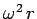. Of course, this acceleration is provided by the gravitational attraction between the satellite and the Earth, which yields an acceleration of magnitude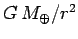. It follows that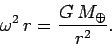(559)

Suppose that the satellite's orbit lies in the Earth's equatorial plane. Moreover, suppose that the satellite's orbital angular velocity just matches the Earth's angular velocity of rotation. In this case, the satellite will appear to hover in the same place in the sky to a stationary observer on the Earth's surface. A satellite with this singular property is known as a geostationary satellite.

Virtually all of the satellites used to monitor the Earth's weather patterns are geostationary in nature. Communications satellites also tend to be geostationary. Of course, the satellites which beam satellite-TV to homes across the world must be geostationary--otherwise, you would need to install an expensive tracking antenna on top of your house in order to pick up the transmissions. Incidentally, the person who first envisaged rapid global telecommunication via a network of geostationary satellites was the science fiction writer Arthur C. Clarke in 1945.

Let us calculate the orbital radius of a geostationary satellite. The angular velocity of the Earth's rotation is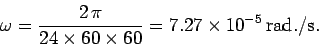(560)

It follows from Eq. (559) that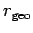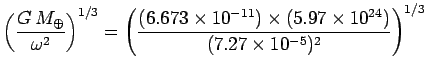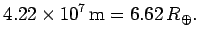(561)

Thus, a geostationary satellite must be placed in a circular orbit whose radius is exactly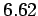times the Earth's radius.Next: Planetary orbits Up: Orbital motion Previous: Gravitational potential energy
Richard Fitzpatrick 2006-02-02